# A chemist is studying the following equilibirum, which has the given equilibrium constant at a certain...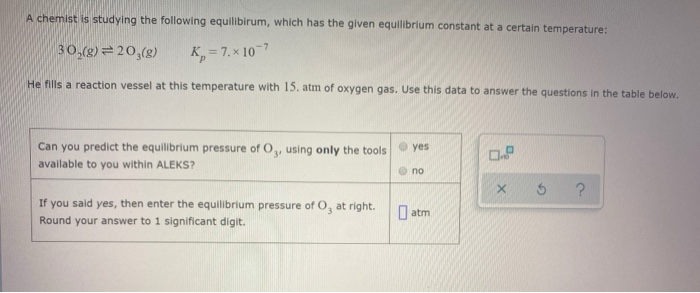A chemist is studying the following equilibirum, which has the given equilibrium constant at a certain temperature: 30,(8) = 20,(8) K = 7.x 10-7 He fills a reaction vessel at this temperature with 15. atm of oxygen gas. Use this data to answer the questions in the table below. yes Can you predict the equilibrium pressure of Oy, using only the tools available to you within ALEKS? no S ? am If you said yes, then enter the equilibrium pressure of O, at right. Round your answer to 1 significant digit.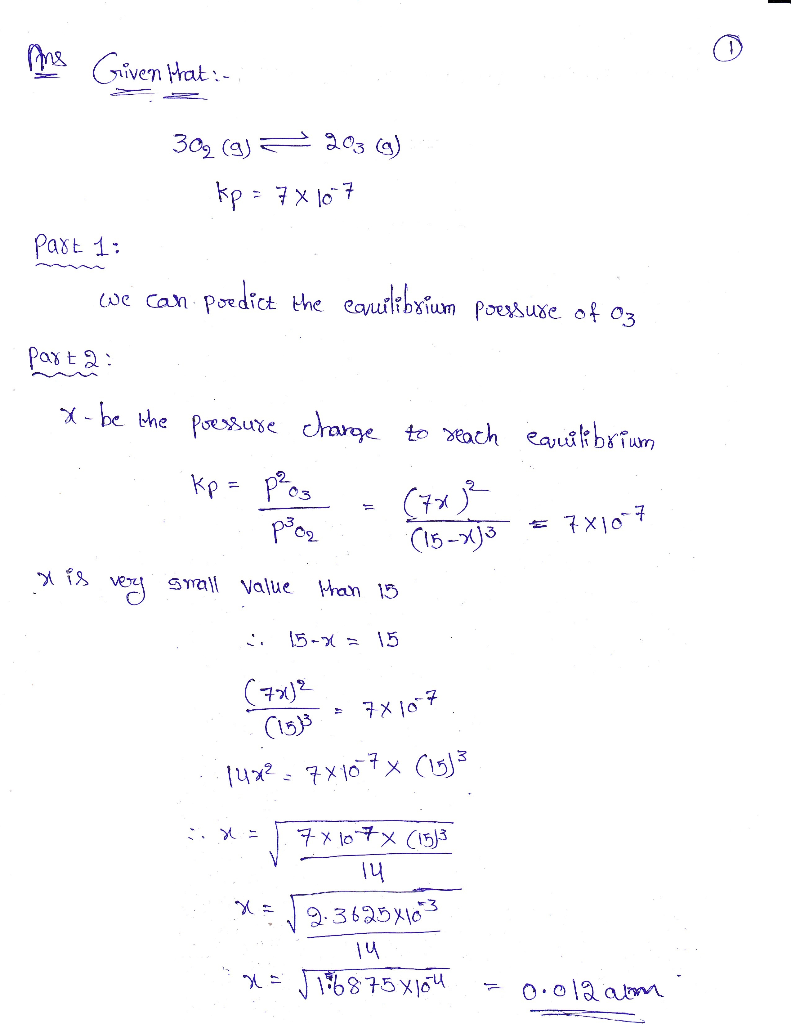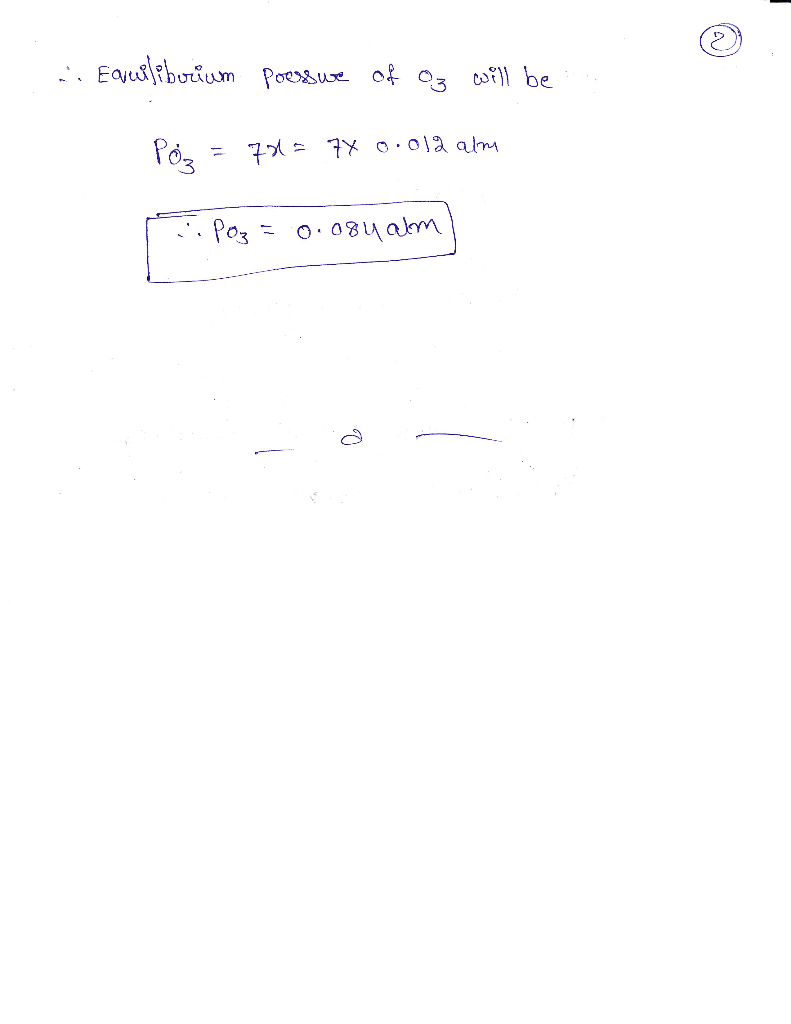#### Earn Coin

Coins can be redeemed for fabulous gifts.

Similar Homework Help Questions
• ### A chemist is studying the following equilibirum, which has the given equilibrium constant at a certain...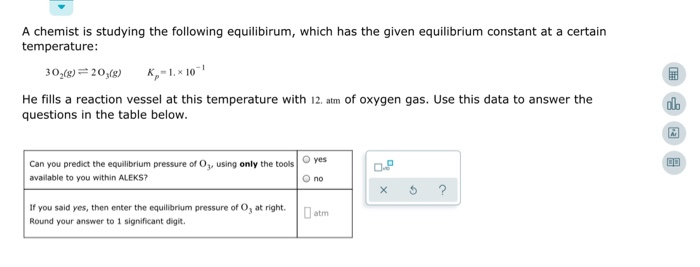A chemist is studying the following equilibirum, which has the given equilibrium constant at a certain temperature: 30,(\$) = 20;(8) K-1, 10 He fills a reaction vessel at this temperature with 12. atm of oxygen gas. Use this data to answer the questions in the table below. Can you predict the equilibrium pressure of Oy, using only the tools | oyes available to you within ALEKS? Ono If you said yes, then enter the equilibrium pressure of O, at right....

• ### A chemist is studying the following equilibirum, which has the given equilibrium constant at a ce...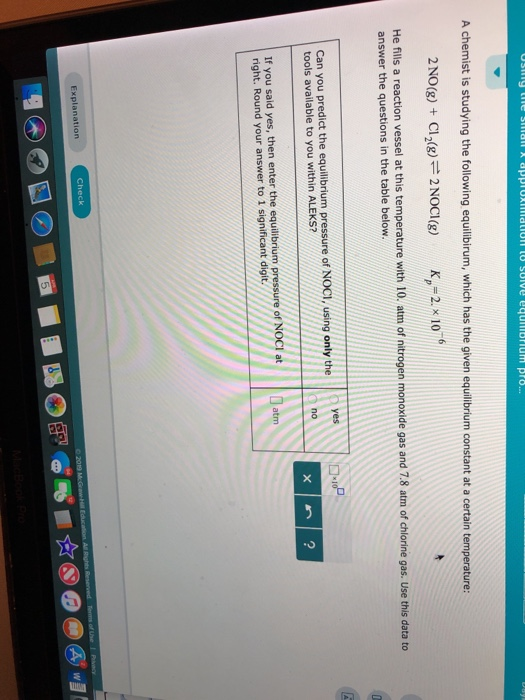A chemist is studying the following equilibirum, which has the given equilibrium constant at a certain temperature: 2NO(g) + C12()2NOCI) 21 He fills a reaction vessel at this temperature with 10. atm of nitrogen monoxide gas and 7.8 atm of chlorine gas. Use this data to answer the questions in the table below. Can you predict the equillbrium pressure of NOCI, using only theYes tools available to you within ALEKS? no If you said yes, then enter the equilibrium pressure...

• ### A chemist is studying the following equilibirum, which has the given equilibrium constant at a certain...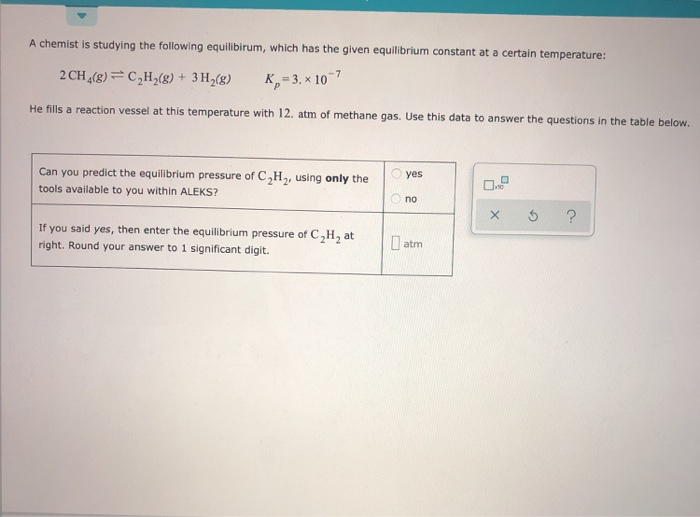A chemist is studying the following equilibirum, which has the given equilibrium constant at a certain temperature: 2 CH4(8) C2H28) + 3H2(g) K 3. x 107 P He fills a reaction vessel at this temperature with 12. atm of methane gas. Use this data to answer the questions in the table below. Can you predict the equilibrium pressure of C,H,, using only the yes tools available to you within ALEKS? no ? X If you said yes, then enter the...

• ### v A chemist is studying the following equilibirum, which has the given equilibrium constant at a...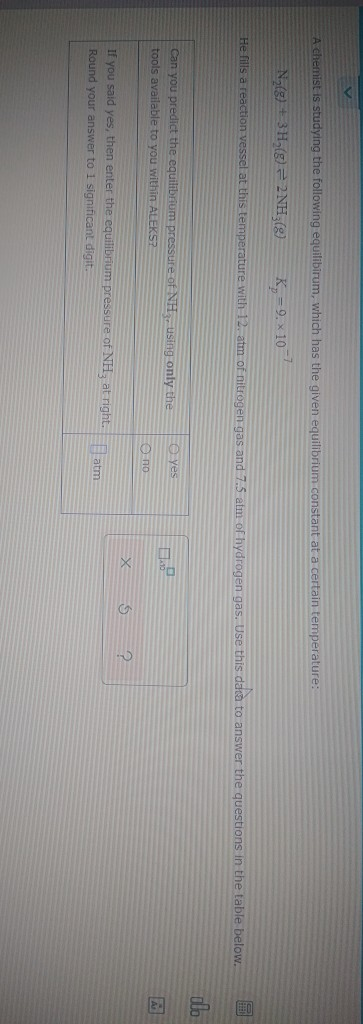v A chemist is studying the following equilibirum, which has the given equilibrium constant at a certain temperature: Na(g) + 3H2(g) = 2 NH3(8) K₂=9. x 10-7 He fills a reaction vessel at this temperature with 12. atm of nitrogen gas and 7.5 atm of hydrogen gas. Use this data to answer the questions in the table below. allo eyes Can you predict the equilibrium pressure of NH, using only the tools available to you within ALEKS? Опо X 6...

• ### A chemist is studying the following equilibirum, which has the given equilibrium constant at a certain...

A chemist is studying the following equilibirum, which has the given equilibrium constant at a certain temperature: N 2 ( g ) + 2 H 2 O ( g ) ⇌ 2 NO ( g ) + 2 H 2 ( g ) ; K p= 5 x 10 ^− 9 He fills a reaction vessel at this temperature with 8.0 atm of nitrogen gas and 13. atm of water vapor. Use this data to answer the questions. enter the...

• ### Calculating an equilibrium constant from a partial equilibrium composition Ammonia will decompose into nitrogen and hydrogen...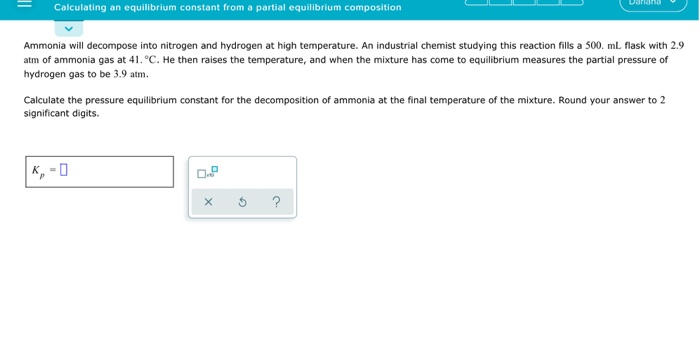Calculating an equilibrium constant from a partial equilibrium composition Ammonia will decompose into nitrogen and hydrogen at high temperature. An industrial chemist studying this reaction fills a 500 ml flask with 2.9 atm of ammonia gas at 41. °C. He then raises the temperature, and when the mixture has come to equilibrium measures the partial pressure of hydrogen gas to be 3.9 atm. Calculate the pressure equilibrium constant for the decomposition of ammonia at the final temperature of the mixture....

• ### A chemist fills a reaction vessel with 9.20 atm nitrogen monoxide (NO) gas, 9.15 atm chlorine (CI) gas, and 7...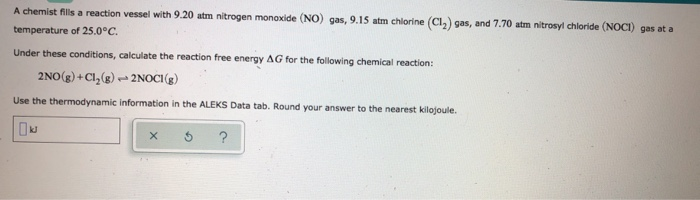A chemist fills a reaction vessel with 9.20 atm nitrogen monoxide (NO) gas, 9.15 atm chlorine (CI) gas, and 7.70 atm nitrosyl chloride (NOCI) gas at a temperature of 25.0°C. Under these conditions, calculate the reaction free energy AG for the following chemical reaction: 2NO(g) +CI (8) - 2NOCI (8) Use the thermodynamic information in the ALEKS Data tab. Round your answer to the nearest kilojoule. X 5 ? A chemist fills a reaction vessel with 9.20 atm nitrogen monoxide...

• ### O ENTROPY AND FREE ENERGY Calculating reaction free energy under nonstandard con... OOOOO Eliza A chemist...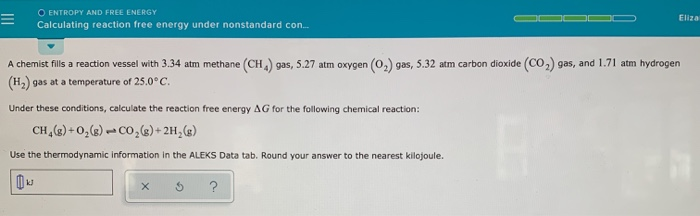O ENTROPY AND FREE ENERGY Calculating reaction free energy under nonstandard con... OOOOO Eliza A chemist fills a reaction vessel with 3.34 atm methane (CH) gas, 5.27 atm oxygen (O2) gas, 5.32 atm carbon dioxide (CO2) gas, and 1.71 atm hydrogen (H) gas at a temperature of 25.0°C. Under these conditions, calculate the reaction free energy AG for the following chemical reaction: CH (8)+02() -00,(\$)+2H (8) Use the thermodynamic information in the ALEKS Data tab. Round your answer to the...

• ### A chemist fills a reaction vessel with 7.72 atm chlorine (C1) gas, 6.29 atm phosphorus (P.)...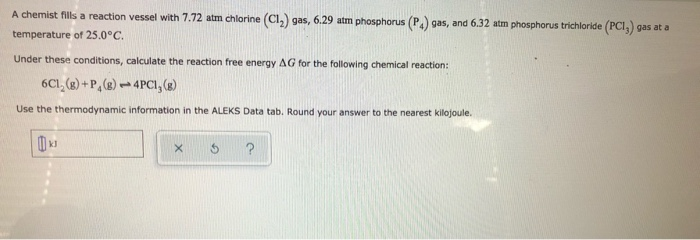A chemist fills a reaction vessel with 7.72 atm chlorine (C1) gas, 6.29 atm phosphorus (P.) gas, and 6.32 atm phosphorus trichloride (PCI) gas at a temperature of 25.0°C. Under these conditions, calculate the reaction free energy AG for the following chemical reaction: 6C1, () +P,(8) 4PC1,(B) Use the thermodynamic information in the ALEKS Data tab. Round your answer to the nearest kilojoule X 5 ?

• ### Ammonia will decompose into nitrogen and hydrogen at high temperature. An industrial chemist stud...

Ammonia will decompose into nitrogen and hydrogen at high temperature. An industrial chemist studying this reaction fills a 5.0 L flask with 1.3 atm of ammonia gas at 46. °C . He then raises the temperature, and when the mixture has come to equilibrium measures the amount of nitrogen gas to be 0.39 atm . Calculate the pressure equilibrium constant for the decomposition of ammonia at the final temperature of the mixture. Round your answer to 2 significant digits. kp=_______